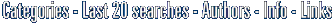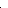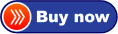# Visual Fractal Fractal software to create Mandelbrot fractal Julia fractal etc. versatileO in short: interesting fractal software you can use Newton's method to solve a complex equation and show the fractal graph in the plot area. wonderful

Easy software similar to Visual Fractal, about:
fractals or free fractal softwareCategoriesVisual Fractal 1.53Click to enlarge

Description:
With this interesting fractal software, you can use Newton's method to solve a complex equation and show the fractal graph in the plot area. Mandelbrot set and Julia set can also be plotted. Graphs created can be saved as bmp files. With this interesting fractal software, you can use Newton's method to solve a complex equation and show the fractal graph in the plot area. Mandelbrot set and Julia set can also be plotted. Graphs created can be saved as bmp files.
Price \$ 25 /Purchase Visual Fractal
Get it Now

Type: Demo
File size: 1289 Kb
Date: 07/23/2010
Homepage
Install support: Install and Uninstall
OS: WinXP
System requirements:
Language: English
Recent changes in this New Release:

Home and Education: Home and Education:Geometry and Mathematic Analysis

 FindGraphFindGraph is a graphing, curve-fitting, and digitizing tool for engineers, scientists and business. Discover the model that best describes your data.

 Visual CalculusVisual Calculus is an easy-to-use calculus grapher for Graphing limit, derivative function, integral, 3D vector, series, ode etc. Which can create 2D, 2.5D and 3D function graphs, animations and table graphs.

 Prime or notSearch, count, save and find primes then prove their primality.Using 4 tools:-The Primality Tester.-Mersenne numbers tester.-The Divisors finder.-The Search engine.-The convertor: Mersenne form-Fermat form-integer-The Splitter...

 Math-Flight 2Learning mathematics can be a challenge for anyone. Math Flight can help you master it with three fun activities to choose from! With lots of graphics and sound effects, your interest in learning math should never decline.

 Visual FractalWith this interesting fractal software, you can use Newton's method to solve a complex equation and show the fractal graph in the plot area.

 Visual ComplexVisual Complex is a graph software to create graph of complex function. 3D function graphs and 2D color maps can be created with this grapher.

 See above information and user's reviews about Visual Fractal Fractal software to create Mandelbrot fractal Julia fractal etc. versatile See also other good software tools: Home and Education: Home and Education:Geometry and Mathematic AnalysisSearch Soft14: Search: the Web within this Web site